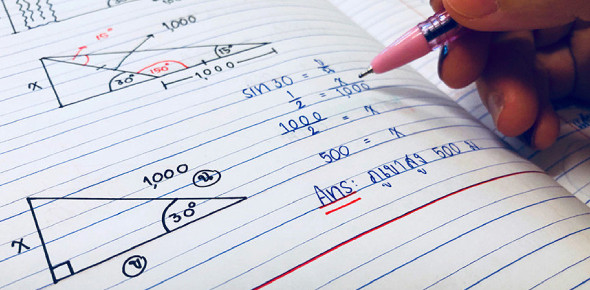Molecular Geometry And Hybridization Quiz #1

10 Questions | Total Attempts: 2202SettingsHybridization is the mixing of atomic orbitals into new hybrid orbitals, suitable for the pairing of electrons. Molecular Geometry highly uses this concept. The quiz below is on the subject. May seem hard, but try it out.

• 1.
According to VSEPR theory, if there are four electron domains in the valence shell of an atom, they will be arranged in a(n) _____________ geometry.
• A.

Octahedral

• B.

Linear

• C.

Tetrahedral

• D.

Trigonal planar

• E.

Trigonal bipyramidal

• 2.
The electron-domain geometry and molecular geometry of iodine trichloride are _____________ and ______________, respectively.
• A.

Trigonal bipyramidal, trigonal planar

• B.

Tetrahedral, trigonal pyramidal

• C.

Trigonal bipyramidal, T-shaped

• D.

Octahedral, trigonal planar

• E.

T-shaped, trigonal planar

• 3.
The molecular geometry of _________ is square planar.
• A.

CCl4

• B.

XeF4

• C.

PH3

• D.

XeF2

• E.

ICl3

• 4.
The electron-domain geometry of a sulfur-centered compound is trigonal bipyramidal. The hybridization of the central sulfur atom is ________.
• A.

Sp

• B.

Sp^2

• C.

Sp^3

• D.

Sp^3d

• E.

Sp^3d^2

• 5.
The hybridization of orbitals on the central atom in a molecule is sp. The electron-domain geometry around this central atom is _________.
• A.

Octahedral

• B.

Linear

• C.

Trigonal planar

• D.

Trigonal bipyramidal

• E.

Tetrahedral

Related TopicsBack to top